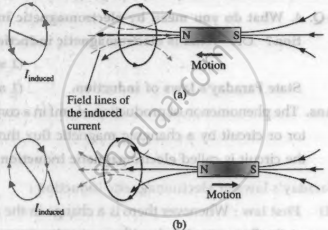# Answer in brief. State and explain Lenz’s law in light of the principle of conservation of energy. - Physics

State and explain Lenz’s law in light of the principle of conservation of energy.

#### Solution

Lenz's law: The direction of the induced current is such as to oppose the change that produces it.

The change that induces a current may be

1. the motion of a conductor in a magnetic field or
2. the change of the magnetic flux through a stationary circuit.

Explanation: Consider Faraday's magnet-and coil experiment. If the bar magnet is moved towards the coil with its N-pole facing the coil, as in the shown first figure, the number of magnetic lines of induction (pointing to the left) through the coil increases. The induced current in the coil sets up a magnetic field of its own pointing to the right (as given by Amperes right-hand rule) to oppose the growing flux due to the magnet. Hence, to move the magnet towards the coil against this repulsive flux of the induced current, we must do work. The work done shows up as electric energy in the coil.When the magnet is withdrawn, with its N-pole still facing the coil, the number of magnetic lines of induction (pointing left) through the coil decreases. The induced current reverses its direction to supplement the decreasing flux with its own, as shown in the second figure. Facing the coil along with the magnet, the induced current is in the clockwise sense. The electric energy in the coil comes from the work done to withdraw the magnet, now against an attractive force. Thus, we see that Lenz's law is a consequence of the law of conservation of energy.

Concept: Lenz's Law
Is there an error in this question or solution?

#### APPEARS IN

Balbharati Physics 12th Standard HSC Maharashtra State Board
Chapter 12 Electromagnetic induction
Exercises | Q 2.2 | Page 286